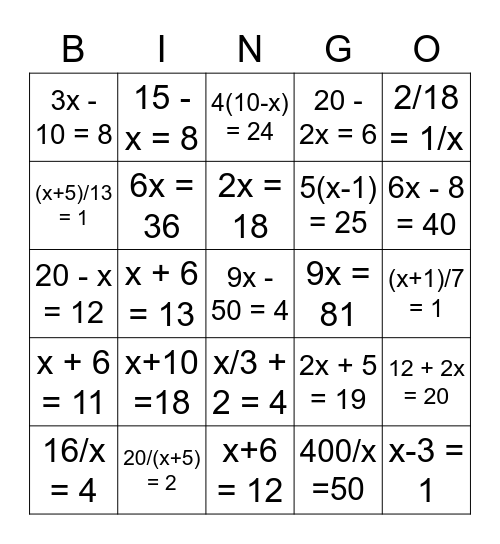# equation BingoThis bingo card has 25 words: x+10 =18, x+6 = 12, 2x = 18, 16/x = 4, 2/18 = 1/x, 3x - 10 = 8, (x+5)/13 = 1, 15 - x = 8, 9x = 81, x/3 + 2 = 4, 5(x-1) = 25, 20 - x = 12, 2x + 5 = 19, 12 + 2x = 20, 6x = 36, x-3 = 1, (x+1)/7 = 1, 4(10-x) = 24, 6x - 8 = 40, x + 6 = 13, 9x - 50 = 4, 20/(x+5) = 2, x + 6 = 11, 20 - 2x = 6 and 400/x =50.

## Play Online